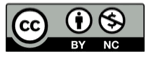# 解决 CSV 文件的第一列不能解析

2023-10-25## 问题描述(Excel 创建的 csv)(Excel 创建的 csv)

### 使用 Python 解析

``````import csv

with open("some.csv", 'r') as f:
print(row["Id"], row["Name"], row["Age"])
``````

``````Traceback (most recent call last):
File "some.py", line 6, in <module>
print(row["Id"], row["Name"], row["Age"])
KeyError: 'Id'
``````

### 使用 Go 解析

``````package main

import (
"encoding/csv"
"fmt"
"io"
"log"
"os"
)

func main() {
f, err := os.Open("some.csv")
if err != nil {
log.Fatalf("Error: %s", err)
}
defer f.Close()

for {
if err == io.EOF {
break
}
if err != nil {
}
for _, field := range record {
if field == "Id" {
fmt.Println(field)
}
}

}
}

``````

## 问题原因## Python 的解决方法

``````import csv

with open("some.csv", 'r', encoding='utf_8_sig') as f:
print(row["Id"], row["Name"], row["Age"])
``````

``````1 Alice 12
2 Bob 8
3 Charlie 10
``````

## Go 语言的解决方法

``````go get -u github.com/dimchansky/utfbom
``````

### 原生 csv 库

``````
package main

import (
"encoding/csv"
"fmt"
"io"
"log"
"os"

"github.com/dimchansky/utfbom"
)

func main() {
f, err := os.Open("some.csv")
if err != nil {
log.Fatalf("Error: %s", err)
}
defer f.Close()

for {
if err == io.EOF {
break
}
if err != nil {
}
for _, field := range record {
if field == "Id" {
fmt.Println(field)
}
}

}
}
``````

## 精彩评论